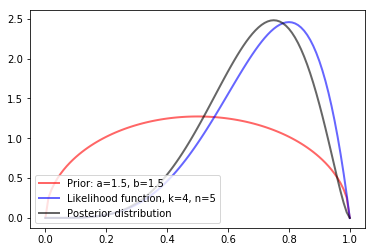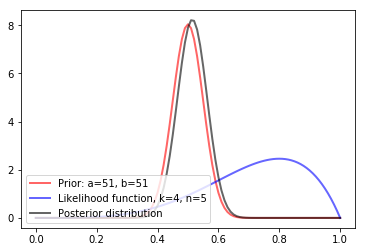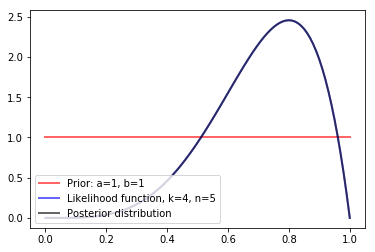# Relation between add-N smoothing and Bayesian inference

### Additive smoothing and Bayesian Inference¶

The additive smoothing approach is an intuitive way to incorporate regularization into statistical data when dealing with counts, by adding hypothetical counts to the observed data. As an example in language modelling, it is common procedure (especially for Naive-Bayes classifiers) to add a word count ("pseudocount") to each word in the vocabulary. This avoids zero probabilities, if -for instance- a word is not observed within a certain class of documents.

Additive smoothing has a direct relation with Bayesian inference, as can be very easily demonstrated in the case of a binomial (counting) process, also known as a coin-flip process. This post discusses how the two are related, which is helpful when having to decide on a prior when dealing with a binomial process.

First, let's define two important distributions.

#### Binomial and beta distributions¶

The binomial distribution is a discrete distribution, that gives the probability of observing $k$ successes after $n$ "coinflip" trials, the coin having a probability of success for each flip equal to $\theta$:

\begin{equation} P(k; n, \theta) \ = \ {k \choose n} \ \theta^k (1-\theta)^{n-k} \end{equation}

The beta distribution is a continuous distribution that is not connected to any process in particular, but is generally used to model the distribution function of a fraction, as it is bounded between 0 and 1. Its probability density function is:

\begin{equation} f_\theta = K \cdot \theta^{a -1} (1-\theta)^{b -1} \end{equation}

The $K$ is a normalization constant and of relevance for the discussion, apart from the fact that its value only depends on the parameters $a$ and $b$, and ensures that the integral over pdf over its domain sums to 1.

Some simple observations:

• switching parameters $a$ and $b$ mirrors the function over $\theta = 0.5$
• therefore, if these are equal, the pdf is symmetric, and the expectation of $\theta is 0.5 • when both parameters are 1, the function is flat and equal to one #### Beta distribution as a conjugate prior for binomial process¶ Let's start with the likelihood function of the binomial function. After observing$m$successes and$n-m$fails, we might want to estimate$\theta$. This is not completely trivial. One option is to maximize the likelihood of the observations given$\theta$:$ P(\mathcal{D} | \theta) $. Choosing the$\theta$that maximizes this function is called the maximum likelihood estimate. The maximum likelihood approach gives, after a single successful coin flip, an estimate for$\theta$of 1, which seems exaggerated if we believe we are in fact dealing with a normal coin. The Bayesian approach also takes a prior distribution into account, which reflects our belief of the values of$\theta$before having seen any data. Without going into the details of Bayes' theorem, the posterior probability (the probability after having made our observations) is proportional to our prior distribution times the likelihood function. Thus: \begin{equation} P(\theta | \mathcal{D}) \propto P(\theta) P(\mathcal{D} | \theta) \ .\end{equation} This measure makes more sense than the likelihood: it tells us the probability of our parameter value, given both our belief and our observations$\mathcal{D}$. If we take a beta function as our prior distribution, multiplying with the likelihood for the binomial parameter$\theta$results in another beta function: \begin{equation} P(\theta) P(\mathcal{D} | \theta) = K \cdot \theta^{(a -1 +k)} \ (1-\theta)^{(b -1 + n -k)} \end{equation} Because of this property, we call the beta distribution a conjugate prior for the binomial distribution. The data (observations) modify the parameters of the prior distribution, but the family of the distribution remains the same. An important observation is that the likelihood function for a binomial process, \begin{equation} \ P(\mathcal{D} | \theta) \propto \theta^k (1-\theta)^{n-k} \ \, \end{equation} is exactly our beta distribution, with$a - 1 = k$and$b - 1 = n - k +1$(the normalization requires that the constant$K$is identical to the binomial coefficient). This means that, following the Bayesian approaches with parameters$a$and$b$for our prior, is the same thing as adding$a-1$successes and$b-1$fails to the observations (which is adding two pseudocounts -one to the successful outcome and one to the failed outcome-, in the case of$a = b = 2$). ##### Example: add-1 smoothing¶ In : from scipy.stats import beta import matplotlib.pyplot as plt import numpy as np def posterior_plotter(a, b, k, n): fig, ax = plt.subplots(1, 1, figsize=(8, 4)) x = np.linspace(beta.ppf(0, a, b), beta.ppf(1, a, b), 101) ax.plot(x, beta.pdf(x, a, b), 'r-', lw=2, alpha=0.6, label='Prior: a={a}, b={b}'.format(a=a, b=b)) ax.plot(x, beta.pdf(x, k+1, n-k+1), 'b-', lw=2, alpha=0.6, label='Likelihood function, k={k}, n={n}'.format(k=k, n=n)) ax.plot(x, beta.pdf(x, a+k, b+n-k), 'k-', lw=2, alpha=0.6, label='Posterior distribution') plt.legend(loc=3);  Example 1: add-one smoothing (beta(1, 1) prior), 4 successes of 5 trials. Because the prior is wide -or, in other words, because we have only 1 pseudocount, equally divided over success and fail-, the prior pulls the posterior only a bit to the middle. In : posterior_plotter(1.5, 1.5, 4, 5)Example 2: a narrow prior (100 pseudocounts, 50 for each outcome), not allowing the data to pull the posterior away from it. In : posterior_plotter(51, 51, 4, 5)Example 2: a flat prior (no pseudocounts). The prior does not affect the posterior. In : posterior_plotter(1, 1, 4, 5)#### Final remarks¶ We saw two different probability functions: the likelihood (purely data-based) and the posterior probability, which also takes into account some prior belief. Point estimates were not discussed, but that is a choice that needs to be made. A convenient choice is often the mode (known as maximum a-posteriori, or MAP), or the mean posterior. The MAP is convenient because it is easily analytically evaluated by searching for the point where the derivative of the (log-) probability is zero. An other, perhaps more common setting than the binomial one, is the multinomial distribution, as encountered for text. The same concept applies here: we add some pseudocount$\alpha$(between 0 and 1) to each possible outcome, and add D*$\alpha\$ to the denominator when calculating the word-probabilities, to ensure a total probability of one.

Deciding an appropriate prior (especially its variance) is often not easy. But in the bi- or multinomial case, thinking in pseudocounts is helpful to easily quantify the effect of the prior.